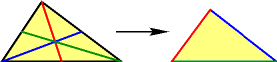# Median TrianglesIf T is a triangle let M(T) be the triangle formed by the three medians of T. (In other words, form the triangle M(T) using the 3 line segments which each start from a vertex of T and end at the center of the opposite side.) What is the relationship of M(M(M(M(T)))) to T?